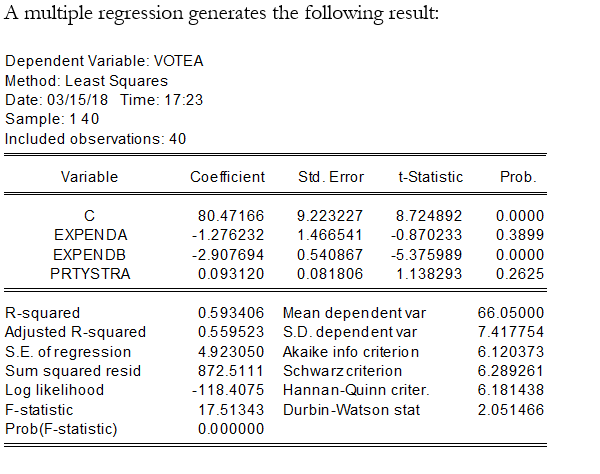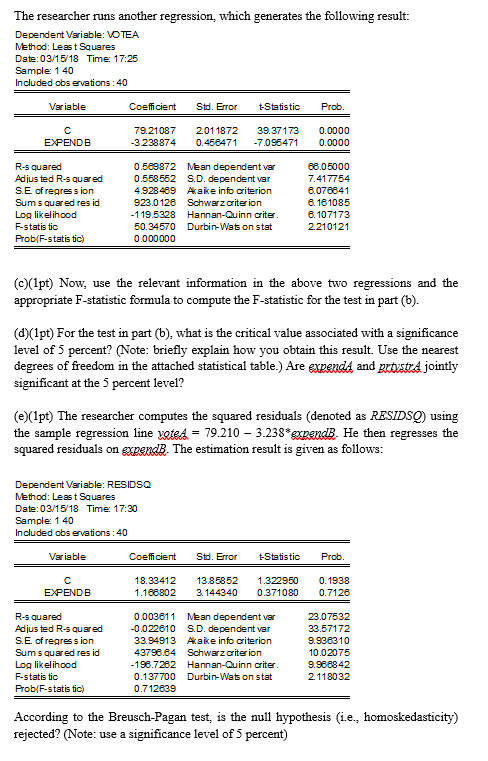# Hi just need some help with questions c, d and e. This are the solutions for parts A and B which …

Hi just need some help with questions c, d and e. This are the solutions for parts A and B which will be needed for solving c, d and e, Thanks very much in advance. (NOW UPDATED) Sorry

Part A and B regression:

The variables are defined as follows:

voteA = percentage of the vote received by Candidate A

expendA =campaign expenditure by Candidate A (in million dollars)

expendB =campaign expenditure by Candidate B (in million dollars)

prtystrA =a measure of party strength for candidate A (the fraction of the most recent presidential vote that went to Aâ€™s party, expressed in percent)A) What is the interpretation of the coefficients? Are the slope coefficients individually significant? (Note: use a significance level of 5 percent).

Coefficient of EXPENDA shows that there is a negative relationshipe between percentage of votes recieved by A and expenditure on campaign by A. if EXPENDA increases by 1 unit percentage of votes received by A decreases by 1.28 units.

Coefficient of EXPENDB shows that there is a negative relationship between  percentage of votes recieved by A and expenditure on campaign by B. If EXPENDB increases by 1 unit percentage of votes received by A decreases by 2.91.

Coeffcient of PRTYSTRA shows that there is a posiitve relationship between percentage of votes received by A and measure of party strength for A. If party strength increases by 1 unit, percentage of votes received by A increases by 0.09 units.

Coefficient is significant if its absolute t statistic is greater than critical t statistic of 1.96. Only EXPENDB has t statistic greater than 1.96. So only EXPENDB is statistically significant.

B) We are interested in conducting a joint significance test on expendA and prtystrA. Write down the null and alternative hypotheses of this test. What are the degrees of freedom associated with this test?

let coefficient of expendA be b2 and coefficient of prtystraA be b3.

H0: b2=b3=0

H1: b2 not equal to b3 not equal to 0

degrees of freedom for numerator is 2 which is the number of restriction.

degrees of freedom for denominator is n-k-1 where n is 40, k is the no. of explanatory variables which is 3. degrees of freedom for denominator is 40-3-1= 36.multiple regression generates the following result: Dependent Variable: VOTEA Method: Least Squares Date: 03/15/18 Time: 17:23 Sample: 1 40 Included observations: 40 Variable Coefficient Std. Error t-Statistic Prob EXPENDA EXPENDB PRTYSTRA 80.47166 9.2232278.7248920.0000 1.276232 .466541 -0.870233 0.3899 2.9076940.540867-5.375989 0.0000 0.093120 0.081806 1.138293 0.2625 R-squared Adjusted R-squared S.E. of regression Sum squared resid Log likelihood F-statistic Prob(F-statistic) 0.593406 0.559523 4.923050 872.5111 Mean dependent var S.D. dependent var Akaike info criterion Schwarz criterion 66.05000 7.417754 6.120373 6.289261 6.181438 2.051466 -118.4075 Hannan-Quinn criter 17.51343 Durbin-Watson stat 0.000000